## Example Questions

### Example Question #122 : Trigonometry

If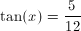, then what is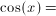?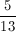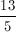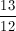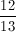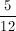Explanation:

If,

and being that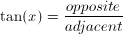,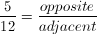.

From this, you can construct a right triangle where 5 is the opposite side to the reference angle and 12 is the adjacent side to the reference angle.

After constructing this triangle, you can then proceed to apply the Pythagorean Theorem.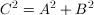As 5 and 12 are the legs of the constructed right triangle, 5 and 12 areandin no particular order. Hence: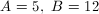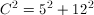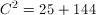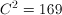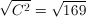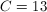We have calculated the hypotenuse of our right triangle. With this knowledge, we can now assign a value to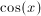. As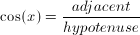, our values of adjacent (12) and our hypotenuse (13) will now be very useful.

The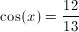.

A helpful pneumonic to remember trig functions: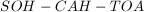. (Sound it out as phonetically as you can)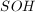: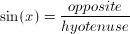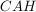: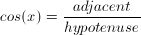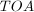: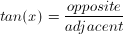### Example Question #123 : Trigonometry

Find the length ofin the right triangle below to the nearest tenth.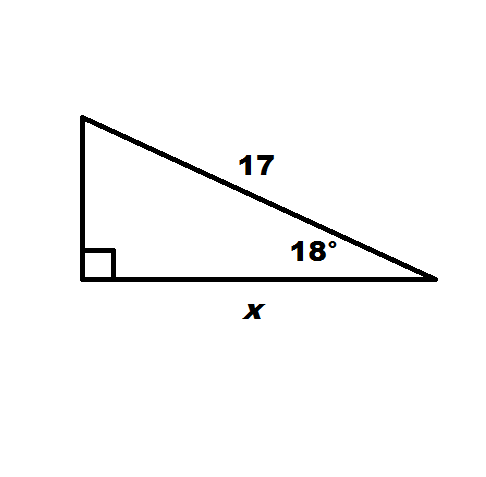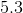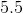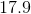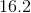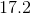Explanation:

Finding a missing side in a right triangle generally utliizes one of two concepts:  the Pythagorean Theorem or trigonometry.  When we have two sides given and need the third side, we use the Pythagorean Theorem.  When we only have one side and need a second but do have the help of an angle other than the right angle, we can use trigonometry.  That is the case our problem falls into.

The three sides of every right triangle can be identified in terms of one of the non-right angles.  The hypotenuse is always the side opposite the right angle (and the longest side).  But if we take one of the non-right angles as our starting point, one of the legs is opposite the angle, and another is adjacent to the angle.  For example, in our triangle, from the starting point of the the 18-degree angle, our hypotenuse is the side of length 17, the adjacent leg is the side labeled, and the unlabeled side is the opposite side.

Given these distinctions, right triangle trigonometry hinges on three ratios:  sine, cosine, and tangent.  Sine is the ratio of the opposite leg to the hypotenuse.  Cosine is the ratio of the adjacent leg to the hypotenuse.  Tangent is the ratio of the opposite leg to the adjacent leg.

Our goal is to find, the length of the adjacent leg.  We know the length of the hypotenuse is 17.  Therefore, our ratio of choice is cosine.  Cosine of our angle (18 degrees) is the ratio ofto 17.  We can write this as an equation.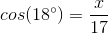Fortunately for us living in our technological age, most calculators have buttons for these three ratios.  But before you can use your calculator, you have to be sure it is in the proper mode.  The calculator needs to be in degree mode, but the way to check and/or change modes depend on the calculator, so if you don't know how to do this, check the manual or consult a knowledgeable person.

But with a calculator in degree mode we can continue.  For most calculators, simply hit the cosine button (abbreviated "cos"), followed by the angle measure (18), and then hit the equal button.

You should get 0.9510565.  That means we get the following equation.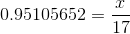From there we have an easy equation to solve.  We simply multiply both sides of the equation by 17.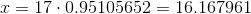Rounded to the nearest tenth, our answer is 16.2.

### Example Question #124 : Trigonometry

The following figure is not drawn to scale.

Using cosine, find the measure of the missing side labeled as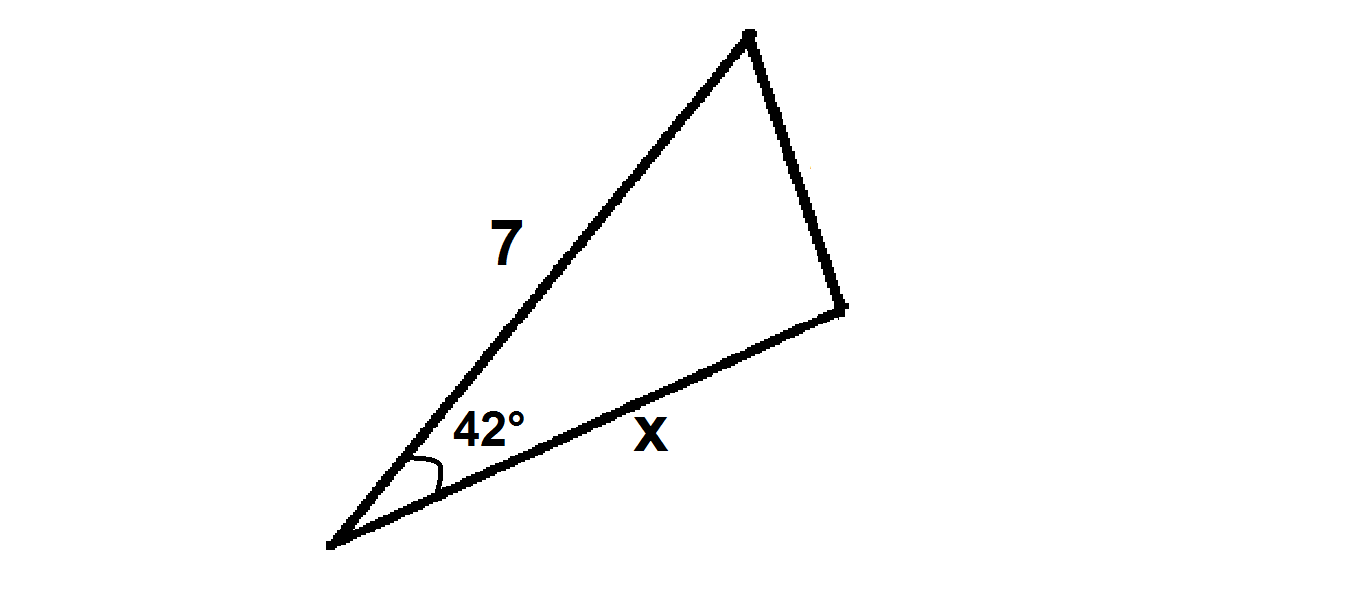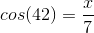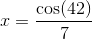Not possible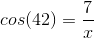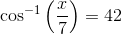Not possible

Explanation:

Trig functions are only possible to use when the triangle is a right triangle. Although the figure may provide ample information and look like it is a right triangle, the latter cannot be assumed because there is no indication of a right angle. It lacks the notation that may be observed in the following: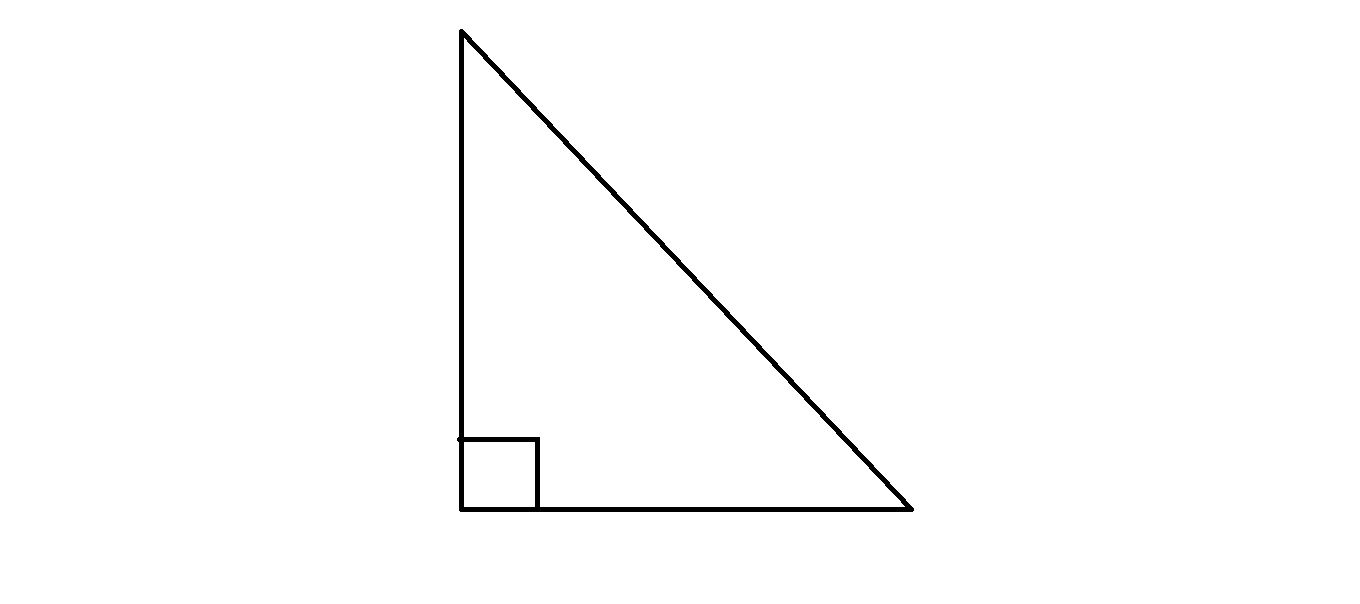Therefore the correct answer to this problem is that it is not possible using a Trigonometric function.

### Example Question #125 : Trigonometry

If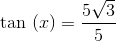, what is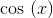?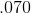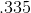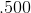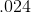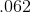Explanation:

SOHCAHTOA tells us that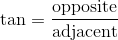. Since this is true, we know that our right triangle has sidesand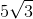. With that as the case, we can use the Pythagorean Theorem to figure out the hypotenuse.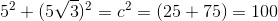Thus,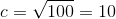. Now, we can calculate cosine using SOHCAHTOA again.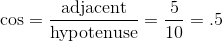.

Thus,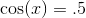.

### Example Question #126 : Trigonometry

If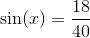, what is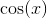?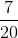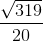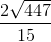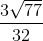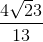Explanation:

SOHCAHTOA tells us that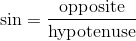. Since this is true, we know that our right triangle has one sideand hypotenuse. With that as the case, we can use the Pythagorean Theorem to figure out the hypotenuse.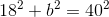--->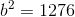Thus,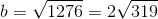. Now, we can calculate cosine using SOHCAHTOA again.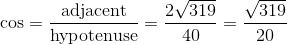.

Thus,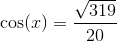.